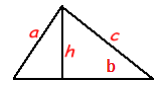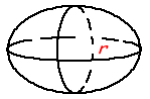Search

“Geometry is the archetype of the beauty of the world.” To understand the spatial skills, starting with nothing is better than geometry. So, let’s initiate to develop these skills by understanding the real-world situations of analyzing shapes and finding figure values.## Introduction:

Spatial and geometric reasoning is not a single ability, skill, or process. By building on strengths and interests that are already present, we can foster an ongoing enthusiasm for mathematics and provide a logical context to develop the number of ideas of the children.

## Theory:

• Perimeter: It is the distance around the outside of the shape. Perimeter is measured in linear units

• Area: It is the space inside the flat shape. Area is measured in square units

• Circumference: It is the distance around the circle. Circumference is measured in linear units

• Volume: It is the amount of space displaced by a solid 3D shape. Volume is measured in cubic units

• Surface Area: It is the total area that the surface of 3D shape occupies. Surface area is measured in square units

SHAPE FORMULASRectangle: Area = length x width = lw Perimeter = 2 x length + 2 x width = 2l + 2wTriangle: Area = ½ x base x height = ½ bh Perimeter = Sum of all sides = a + b + cCircle: r: Radius: distance from the center to a point on the circle d: diameter: the distance between two points on the circle through the center Circumference = 2𝜋r = 𝜋d (Assume 𝜋 = 3.14)

Dimensional ShapesCylinder: Volume = 𝜋r2 x height = 𝜋r2h (Assume 𝜋 = 3.14) Surface = 2𝜋rh + 2𝜋r2 (Assume 𝜋 = 3.14)Cone: Volume = ⅓ 𝜋r2h (Assume 𝜋 = 3.14) Surface = 𝜋r(r + h2 + r2 ) (Assume 𝜋 = 3.14)Sphere: Volume = 4/3 𝜋r3 (Assume 𝜋 = 3.14)